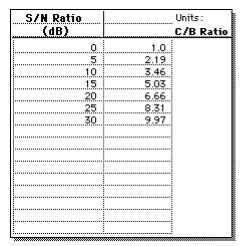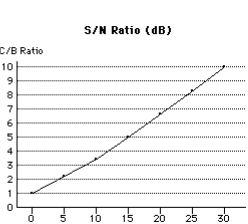Courses

# Shannon's Theorem Electronics and Communication Engineering (ECE) Notes | EduRev

## Communication Theory

Created by: Machine Experts

## Electronics and Communication Engineering (ECE) : Shannon's Theorem Electronics and Communication Engineering (ECE) Notes | EduRev

The document Shannon's Theorem Electronics and Communication Engineering (ECE) Notes | EduRev is a part of the Electronics and Communication Engineering (ECE) Course Communication Theory.
All you need of Electronics and Communication Engineering (ECE) at this link: Electronics and Communication Engineering (ECE)

Shannon's Theorem:

Shannon's Theorem gives an upper bound to the capacity of a link, in bits per second (bps), as a function of the available bandwidth and the signal-to-noise ratio of the link.

The Theorem can be stated as:

C = B * log2(1+ S/N)

where C is the achievable channel capacity, B is the bandwidth of the line, S is the average signal power and N is the average noise power.

The signal-to-noise ratio (S/N) is usually expressed in decibels (dB) given by the formula:

10 * log10(S/N)

so for example a signal-to-noise ratio of 1000 is commonly expressed as

10 * log10(1000) = 30 dB.

Here is a graph showing the relationship between C/B and S/N (in dB):Examples

Here are two examples of the use of Shannon's Theorem.

Modem
For a typical telephone line with a signal-to-noise ratio of 30dB and an audio bandwidth of 3kHz, we get a maximum data rate of:

C = 3000 * log2(1001)

which is a little less than 30 kbps.

Satellite TV Channel
For a satellite TV channel with a signal-to noise ratio of 20 dB and a video bandwidth of 10MHz, we get a maximum data rate of:

C=10000000 * log2(101)

CHANNEL CAPACITY:

In electrical engineering, computer science and information theory, channel capacity is the tightest upper bound on the amount of information that can be reliably transmitted over a communications channel. By the noisychannel coding theorem, the channel capacity of a given channel is the limiting information rate (in units of information per unit time) that can be achieved with arbitrarily small error probability.

Information theory, developed by Claude E. Shannon during World War II, defines the notion of channel capacity and provides a mathematical model by which one can compute it. The key result states that the capacity of the channel, as defined above, is given by the maximum of the mutual information between the input and output of the channel, where the maximization is with respect to the input distribution.

BANDWIDTH:

It has several related meanings:

• Bandwidth (signal processing) or analog bandwidth, frequency bandwidth or radio bandwidth: a measure of the width of a range of frequencies, measured in hertz
• Bandwidth (computing) or digital bandwidth: a rate of data transfer, bit rate or throughput, measured in bits per second (bps)
• Spectral line width: the width of an atomic or molecular spectral line, measured in hertz

Bandwidth can also refer to:

• Bandwidth (linear algebra), the width of the terms around the diagonal of a matrix hypotenuse
• In kernel density estimation, "bandwidth" describes the width of the convolution kernel used
• A normative expected range of linguistic behavior in language expectancy theory
• In business jargon, the resources needed to complete a task or project
• Graph bandwidth, in graph theory
Offer running on EduRev: Apply code STAYHOME200 to get INR 200 off on our premium plan EduRev Infinity!

,

,

,

,

,

,

,

,

,

,

,

,

,

,

,

,

,

,

,

,

,

;